GRE Subject Test: Chemistry : Molecular and Electronic Geometries

Example Questions

Example Question #1 : Vsepr Geometry

Which of the following is not the correct geometric configuration for the given molecule?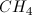, tetrahedral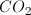, linear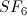, trigonal bipyramidal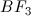, trigonal planar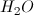, bent, trigonal bipyramidal

Explanation:

Recall the following relationships between geometry and number of pairs of electrons on the central atom.

2: linear

3: trigonal planar

4: tetrahedral

5: trigonal bipyriamidal

6: octahedral

To visualize the geometry, we need to think of how many electron pairs are on the central atom. Drawing Lewis dot diagrams may be helpful here. None of the answer choices has lone central electron pairs, with the exception of water, so the number of atoms bound to the central atom is the same as the number of central electron pairs.

The only one that does not match up with the correct geometry is SF6, which is actually octahedral since it has six central electron pairs. In a water molecule, the central oxygen has six valence electrons, plus one from each bond with hydrogen, for a total of eight central electrons and four central electron pairs. So, this geometry is a variation on the tetrahedral form (bent), in which two central electron pairs are not bound.

Example Question #2 : Diagrams And Geometry

Which of the following molecules exhibits a trigonal pyramidal geometry?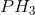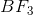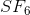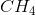Explanation:

The trigonal pyramidal geometry is implemented by molecules in which the central atom has three atoms and a lone pair attached.has three hydrogens attached to the central phosphorus as well as a lone pair, which can be determined by drawing the Lewis structure of the molecule. As a result, it has trigonal pyramidal geometry.is trigonal planar.is tetrahedral.is octahedral.

Example Question #1 : Molecular And Electronic Geometries

Which of the following molecules has an electronic geometry that is the same as its molecular geometry?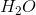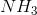Explanation:

Electronic and molecular geometries are only the same when there are no lone pairs around the central atom in the molecule.is the only given option that does not have a lone pair on the central atom, so the electronic geometry is the same as the molecular geometry (in this case, tetrahedral).

Example Question #181 : Ap Chemistry

What is the molecular shape of?

Trigonal planar

Tetrahedral

Trigonal pyramidal

Trigonal bipyramidal

Octahedral